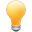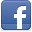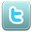# TypeScript Closures Tutorial with Examples

#### View more Tutorials:Follow us on our fanpages to receive notifications every time there are new articles.FacebookTwitter

## 1- What is Closure?

In the TypeScript programming language, a Closure is a special function.
• Similar to a function, a Closure is a block of statements with parameters and can return a value or nothing.
• Unlike a function, a closure has no name. However, you can identify it through a variable.
Syntax:
``````
// Closure syntax
function(arg1: data_type1, arg2: data_type2, argN: data_typeN) {
// Statements
}
// Declare a variable as an alias for Closure
var alias = function(arg1: data_type1, arg2: data_type2, argN: data_typeN) {
// Statements
};
``````
The example below shows the difference between a function and a closure. Conclusion: a closure is a function without a name.
``````
// This is a function called sayHello
function sayHello(name: string) {
console.log(`Hello \${name}`);
}
// This is a closure (A function without name)
function (name: string) {
console.log(`Hello \${name}`);
}
``````
Basically, if you create a Closure that you don't use immediately, you must identify it, or else the compiler will report an error. For example:
Closure ID Example
``````
// Compile Error!!!!!
function(a: number, b: number) {
return a + b;
}
// OK
var closureId = function(a: number, b: number) {
return a + b;
}
``````
You may not need an identifier for a Closure if you create it and call it directly.
closure_ex1.ts
``````
// Create a Closure and call it directly!
(function (name: string) {
console.log(`Hello \${name}`);
})('Tom');

// --- Equivalent to: ---

// Identifier for a closure:
var sayHello = function (name: string) {
console.log(`Hello \${name}`);
};
// Call closure via identifier:
sayHello('Tom');
``````
Another example:
closure_ex2.ts
``````
// Create a Closure and call it directly!
var result1 = (function (a: number, b: number) {
return a + b;
})(100, 200);

// --- Equivalent to: ---

// Identifier for a closure:
var sum = function (a: number, b: number) {
return a + b;
};
// Call closure via identifier:
var result2 = sum(100, 200);
``````

## 2- Arrow syntax

TypeScript allows you to declare a Closure using the arrow syntax, which is also commonly known as Lambda syntax. Compared to the traditional syntax, the arrow syntax omits the function keyword and adds an arrow ( => ) between the parameter block and the content block.
``````
// Arrow Syntax (Lambda Syntax):
(arg1: data_type1, arg2: data_type2, argN: data_typeN) => {
// Statements
}

function(arg1: data_type1, arg2: data_type2, argN: data_typeN)  {
// Statements
}
``````
Example:
closure_arrow_ex1.ts
``````
// Create a Closure with Arrow Syntax.
var introduction = (name: string, country?: string) => {
if (country) {
console.log(`Hello, My name is \${name} from \${country}`);
} else {
console.log(`Hello, My name is \${name}`);
}
};
// Call the Closure
introduction('Tom', 'USA');

// --- Equivalent to: ----

// Create a Closure with Arrow Syntax and call it directly:
((name: string, country?: string) => {
if (country) {
console.log(`Hello, My name is \${name} from \${country}`);
} else {
console.log(`Hello, My name is \${name}`);
}
})('Tom', 'USA');
``````
If the Closure's content consists of only one expression, you can write it more succinctly:
``````
var closureId1 =  (arg1: data_type1, arg2: data_type2, argN: data_typeN)  => {
console.log('Something');
}
// --- Equivalent to: ----
var closureId1 =  (data_type1 arg1, data_type2 arg2, data_typeN argN) => console.log('Something');

var closureId2 =  function(arg1: data_type1, arg2: data_type2, argN: data_typeN) => {
return a_value;
}
// --- Equivalent to: ----
var closureId2 =  function(arg1: data_type1, arg2: data_type2, argN: data_typeN) => a_value;
``````
Example:
closure_arrow_ex2.ts
``````
// Create a Closure
var minus = (a: number, b: number) => a - b;

var result = minus(100, 200); // Call a closure.
console.log(`result = \${result}`);

// Create a Closure
var sayBye = (name: string) => console.log(`Bye \${name}`);
sayBye('Tom'); // Call a closure.
``````
Output:
``````
result = -100
Bye Tom
``````

## 3- Define a function type

In TypeScript, the type keyword is used to define a new data type. In this section we will discuss how to use the type keyword to define a function type (Also called a Closure type).
Syntax:
``````
type Function_Type_Name = (arg1: data_type1, arg2: data_type2, argN: data_typeN) => return_type;
``````
Example: Define a function type that accepts a parameter of number type and returns a value of number type:
function_type_ex1.ts
``````
// A Function Type:
type TaxCalculator = (amount: number) => number;

function function_type_ex1_test()  {
var usTaxCalculator: TaxCalculator = (amount: number) =>  {
return amount * 10 / 100;
};
var vnTaxCalculator: TaxCalculator = (amount: number) =>  amount * 5 / 100;

var caTaxCalculator: TaxCalculator = function(amount: number)  {
return amount * 8 / 100;
}
const AMOUNT = 1000;
var usTaxAmount = usTaxCalculator(AMOUNT);
var vnTaxAmount = vnTaxCalculator(AMOUNT);
var caTaxAmount = caTaxCalculator(AMOUNT);

console.log(`Tax amount according to US calculation: \${usTaxAmount}`);
console.log(`Tax amount according to Vietnam calculation: \${vnTaxAmount}`);
console.log(`Tax amount according to Canada calculation: \${caTaxAmount}`);
}
function_type_ex1_test(); // Call the function.
``````
Output:
``````
Tax amount according to US calculation: 100
Tax amount according to Vietnam calculation: 50
Tax amount according to Canada calculation: 80
``````

## 4- Function type in parameter

Function Type can appear as a parameter of another function, closure, method, or constructor.
function_type_in_args_ex1.ts
``````
// Define a Function Type:
type MyTaxCalculator = (value: number) => number;

function calculateTaxAmount1(amount: number, calculator: MyTaxCalculator) {
var taxAmount = calculator(amount);
console.log(`Tax Amount: \${taxAmount}`);
}
// --- Equivalent to: ---

function calculateTaxAmount2(amount: number, calculator: (value: number) => number) {
var taxAmount = calculator(amount);
console.log(`Tax Amount: \${taxAmount}`);
}
``````
Example:
function_type_in_args_ex2.ts
``````
// A Function accepts 2 parameters:
// 1 - amount: number
// 2 - calculator : (number) => (number)
function printTaxAmount(amount: number, calculator: (value: number) => number) {
var taxAmount = calculator(amount);
console.log(`Tax Amount: \${taxAmount}`);
}
function function_type_in_args_ex2_test() {
// Function Type: (number) => (number)
var usTaxCalculator = (value: number) => {
return value * 10 / 100;
};
// Function Type: (number) => (number)
var vnTaxCalculator: TaxCalculator = (value: number) => value * 5 / 100;

printTaxAmount(1000, usTaxCalculator);
printTaxAmount(1000, vnTaxCalculator);
printTaxAmount(1000, (value: number) => value * 8 / 100);
}
function_type_in_args_ex2_test(); // Call the function.
``````
Output:
``````
Tax Amount: 100
Tax Amount: 50
Tax Amount: 80
``````

#### View more Tutorials:

Maybe you are interested

These are online courses outside the o7planning website that we introduced, which may include free or discounted courses.

•AngularJS Foundation: From 0 to Hero
•Bestseller.How to build persistence mongoDB app with Go
•AWS Serverless APIs & Apps - A Complete Introduction
•Learn Bootstrap 4 The Complete Guide by Building 8 Projects
•Master Computer Vision™ OpenCV4 in Python with Deep Learning
•PHP & MySQL For Beginners
•Struts 2 Framework for Beginners
•ReactJS and Flux: Learn By Building 10 Projects
•Java for Intermediate Users
•Starting 2D Game Development in Unity with C#
•Complete CodeIgniter 3 Series with Bootstrap 4 + Projects
•iOS 11 Programming with SWIFT
•Expert Ruby on Rails 5
•Complete Step By Step Java For Testers
•Java Persistence: Hibernate and JPA Fundamentals
•Learning Path: Bootstrap: The Complete Guide to Bootstrap 4
•Projects in Android
•jQuery 2018 and beyond course
•Build a Real Time Chat App with JavaScript and Firebase
•Create Android and iOS App using HTML, CSS and JS
•The Complete Flutter UI Masterclass | iOS & Android in Dart
•AWS CloudFormation Master Class
•HTML5 and CSS3 For Beginners - A Complete Understanding
•Learn C# with ASP.NET and SQL Server
•Understanding JDBC with PostgreSQL (A step by step guide)